# Determining if a point lies on the interior of a polygon

Written by Paul Bourke 1997 (see the original site in Australia)

### Solution 1 (2D)

The following is a simple solution to the problem often encountered in computer graphics, determining whether or not a point (x,y) lies inside or outside a 2D polygonally bounded plane. This is necessary for example in applications such as polygon filling on raster devices. hatching in drafting software, and determining the intersection of multiple polygons.

Consider a polygon made up of N vertices (xi,yi) where i ranges from 0 to N-1. The last vertex (xN,yN) is assumed to be the same as the first vertex (x0,y0), that is, the polygon is closed. To determine the status of a point (xp,yp) consider a horizontal ray emanating from (xp,yp) and to the right. If the number of times this ray intersects the line segments making up the polygon is even then the point is outside the polygon. Whereas if the number of intersections is odd then the point (xp,yp) lies inside the polygon. The following shows the ray for some sample points and should make the technique clear.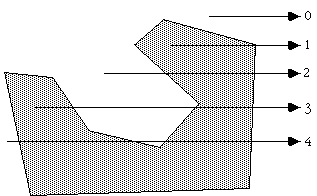Note: for the purposes of this discussion 0 will be considered even, the test for even or odd will be based on modulus 2, that is, if the number of intersections modulus 2 is 0 then the number is even, if it is 1 then it is odd.

The only trick is what happens in the special cases when an edge or vertex of the polygon lies on the ray from (xp,yp). The possible situations are illustrated below.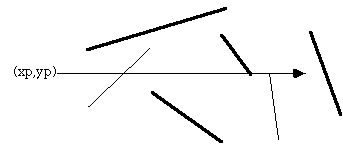The thick lines above are not considered as valid intersections, the thin lines do count as intersections. Ignoring the case of an edge lying along the ray or an edge ending on the ray ensures that the endpoints are only counted once.

Note that this algorithm also works for polygons with holes as illustrated below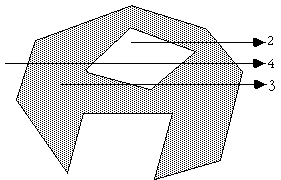The following C function returns INSIDE or OUTSIDE indicating the status of a point P with respect to a polygon with N points.

```#define MIN(x,y) (x < y ? x : y)
#define MAX(x,y) (x > y ? x : y)
#define INSIDE 0
#define OUTSIDE 1

typedef struct {
double x,y;
} Point;

int InsidePolygon(Point *polygon,int N,Point p)
{
int counter = 0;
int i;
double xinters;
Point p1,p2;

p1 = polygon;
for (i=1;i<=N;i++) {
p2 = polygon[i % N];
if (p.y > MIN(p1.y,p2.y)) {
if (p.y <= MAX(p1.y,p2.y)) {
if (p.x <= MAX(p1.x,p2.x)) {
if (p1.y != p2.y) {
xinters = (p.y-p1.y)*(p2.x-p1.x)/(p2.y-p1.y)+p1.x;
if (p1.x == p2.x || p.x <= xinters)
counter++;
}
}
}
}
p1 = p2;
}

if (counter % 2 == 0)
return(OUTSIDE);
else
return(INSIDE);
}```

The following code is by Randolph Franklin, it returns 1 for interior points and 0 for exterior points.

```    int pnpoly(int npol, float *xp, float *yp, float x, float y)
{
int i, j, c = 0;
for (i = 0, j = npol-1; i < npol; j = i++) {
if ((((yp[i] <= y) && (y < yp[j])) ||
((yp[j] <= y) && (y < yp[i]))) &&
(x < (xp[j] - xp[i]) * (y - yp[i]) / (yp[j] - yp[i]) + xp[i]))
c = !c;
}
return c;
}
```

### Solution 2 (2D)

Another solution forwarded by Philippe Reverdy is to compute the sum of the angles made between the test point and each pair of points making up the polygon. If this sum is 2pi then the point is an interior point, if 0 then the point is an exterior point. This also works for polygons with holes given the polygon is defined with a path made up of coincident edges into and out of the hole as is common practice in many CAD packages.

The inside/outside test might then be defined in C as
```typedef struct {
int h,v;
} Point;

int InsidePolygon(Point *polygon,int n,Point p)
{
int i;
double angle=0;
Point p1,p2;

for (i=0;i<n;i++) {
p1.h = polygon[i].h - p.h;
p1.v = polygon[i].v - p.v;
p2.h = polygon[(i+1)%n].h - p.h;
p2.v = polygon[(i+1)%n].v - p.v;
angle += Angle2D(p1.h,p1.v,p2.h,p2.v);
}

if (ABS(angle) < PI)
return(FALSE);
else
return(TRUE);
}

/*
Return the angle between two vectors on a plane
The angle is from vector 1 to vector 2, positive anticlockwise
The result is between -pi -> pi
*/
double Angle2D(double x1, double y1, double x2, double y2)
{
double dtheta,theta1,theta2;

theta1 = atan2(y1,x1);
theta2 = atan2(y2,x2);
dtheta = theta2 - theta1;
while (dtheta > PI)
dtheta -= TWOPI;
while (dtheta < -PI)
dtheta += TWOPI;

return(dtheta);
}
```

### Solution 3 (2D)

There are other solutions to this problem for polygons with special attributes. If the polygon is convex then one can consider the polygon as a "path" from the first vertex. A point is on the interior of this polygons if it is always on the same side of all the line segments making up the path.

Given a line segment between P0 (x0,y0) and P1 (x1,y1), another point P (x,y) has the following relationship to the line segment.

Compute
(y - y0) (x1 - x0) - (x - x0) (y1 - y0)

if it is less than 0 then P is to the right of the line segment, if greater than 0 it is to the left, if equal to 0 then it lies on the line segment.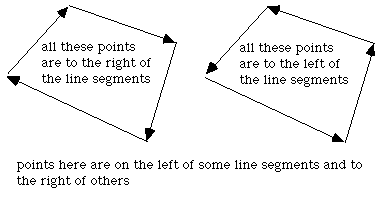### Solution 4 (3D)

 This solution was motivated by solution 2 and correspondence with Reinier van Vliet and Remco Lam. To determine whether a point is on the interior of a convex polygon in 3D one might be tempted to first determine whether the point is on the plane, then determine it's interior status. Both of these can be accomplished at once by computing the sum of the angles between the test point (q below) and every pair of edge points p[i]->p[i+1]. This sum will only be twopi if both the point is on the plane of the polygon AND on the interior. The angle sum will tend to 0 the further away from the polygon point q becomes.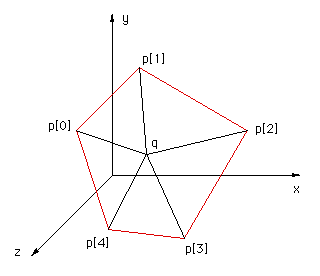The following code snippet returns the angle sum between the test point q and all the vertex pairs. Note that the angle sum is returned in radians.

```typedef struct {
double x,y,z;
} XYZ;
#define EPSILON  0.0000001
#define MODULUS(p) (sqrt(p.x*p.x + p.y*p.y + p.z*p.z))
#define TWOPI 6.283185307179586476925287
#define RTOD 57.2957795

double CalcAngleSum(XYZ q,XYZ *p,int n)
{
int i;
double m1,m2;
double anglesum=0,costheta;
XYZ p1,p2;

for (i=0;i<n;i++) {

p1.x = p[i].x - q.x;
p1.y = p[i].y - q.y;
p1.z = p[i].z - q.z;
p2.x = p[(i+1)%n].x - q.x;
p2.y = p[(i+1)%n].y - q.y;
p2.z = p[(i+1)%n].z - q.z;

m1 = MODULUS(p1);
m2 = MODULUS(p2);
if (m1*m2 <= EPSILON)
return(TWOPI); /* We are on a node, consider this inside */
else
costheta = (p1.x*p2.x + p1.y*p2.y + p1.z*p2.z) / (m1*m2);

anglesum += acos(costheta);
}
return(anglesum);
}
```